Complex NumbersOverview

Expect to see some questions on the topic of Complex Numbers on the SAT. In this section, we will review the following aspects of this topic:

• Definition of a Complex Number
• Complex Multiplication and Division
• Graphing Complex Numbers
• Comparing Complex Numbers
• Closure Under the Complex Number System
• Complex Roots of Equations

Let’s look at each of these in more detail:

Definition of a Complex Number

The real number system consists of quantities that can be graphed on a one-dimensional number line. These are examples of graphs of real numbers.There is another one-dimensional number system that can be graphed on a number line. This is the imaginary number system, and here is such a number line:Each imaginary number is a multiple of i. What is i? It is defined by the following identity:The square root of negative one is not a real number. All numbers that are a multiple of i are imaginary numbers.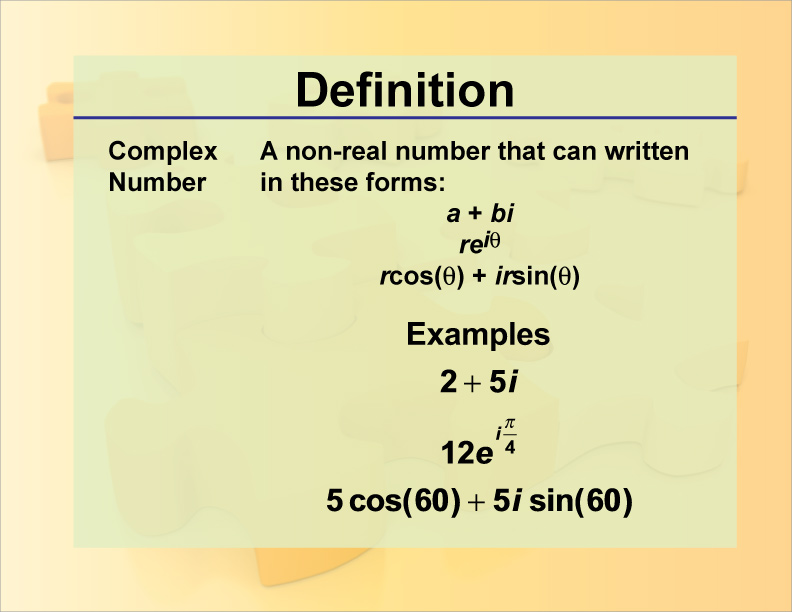We can now combine both number systems to define the complex number system: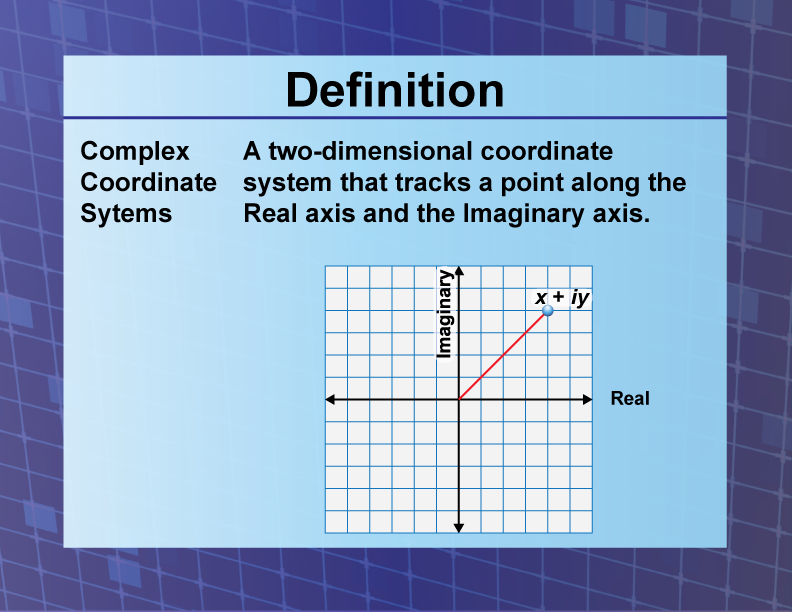Complex numbers are two dimensional and require a two-dimensional coordinate system to graph them. Think of the complex number system as taking the number line of real numbers and using that as the horizontal axis, then taking the number line of imaginary numbers as and using it as the vertical axis.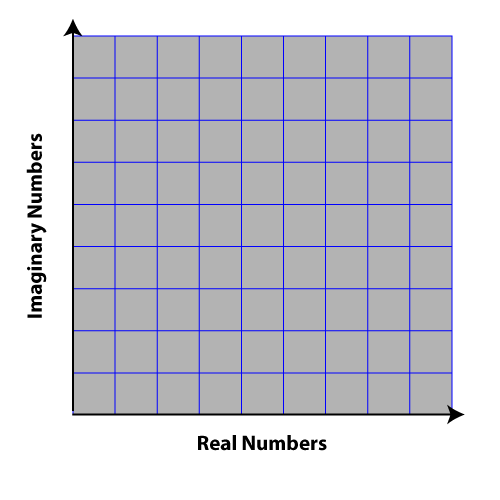A complex number can be written in three different forms, as shown in the definition of a complex number, but for the purposes of the SAT, this is the form to focus on:Note that a complex number has a real number part and an imaginary part. To learn more about complex numbers, click on this link

Properties of Complex Numbers

In this section we go over the properties of complex numbers, including the following:

• Imaginary Numbers to Odd or Even Powers
• Graphs of Complex Numbers
• Complex Conjugates and Their Graphs
• Magnitude of a Complex Number

Imaginary Numbers to Odd or Even Powers

The imaginary number i raised to integer powers alternates from real to imaginary, with alternating signs. Here are the results of raising i to the first five integer powers.Expect SAT Math questions that rely on an understanding of this property of i.

Graphs of Complex Numbers

Since complex numbers are two-dimensional, representing them graphically involves a two-dimensional coordinate system. A complex number of the form a + bi is graphed as if it were a pair of coordinates, (a, b) on the Cartesian system.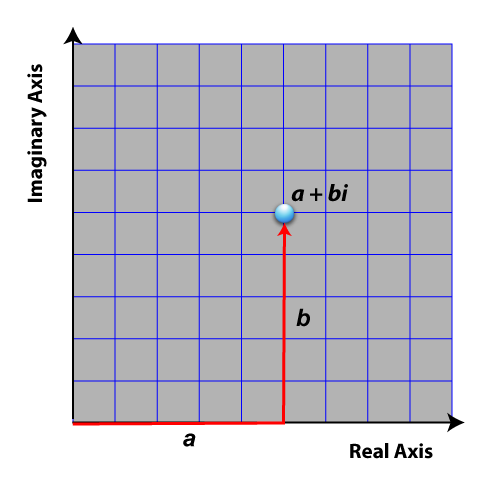The real part, a, is graphed along the horizontal axis. The imaginary part, bi, is graphed along the vertical axis.

To see examples of plotting complex numbers, click on the following link

Complex Conjugates and Their Graphs

Every complex number has what is known as a complex conjugate. This is what such a pair of numbers look like.

 Complex Number Complex ConjugateHere are some examples of complex numbers and their conjugates:

 Complex Number Complex ConjugateThis is what the graph of a complex number and its conjugate look like.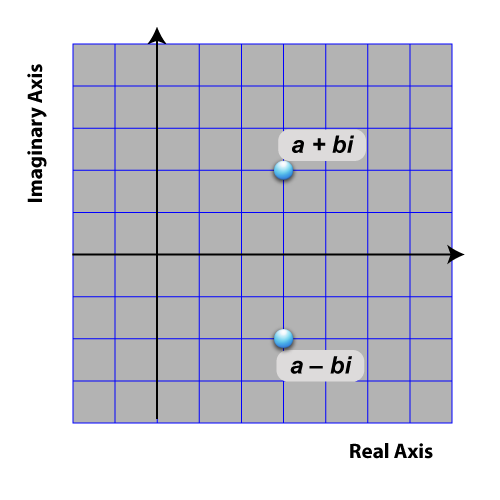Notice that a complex number and its conjugate are mirror images of each other across the horizontal axis.

Magnitude of a Complex Number

Because complex numbers require a two-dimensional coordinate system to represent them graphically, we can measure the distance the number is from the origin.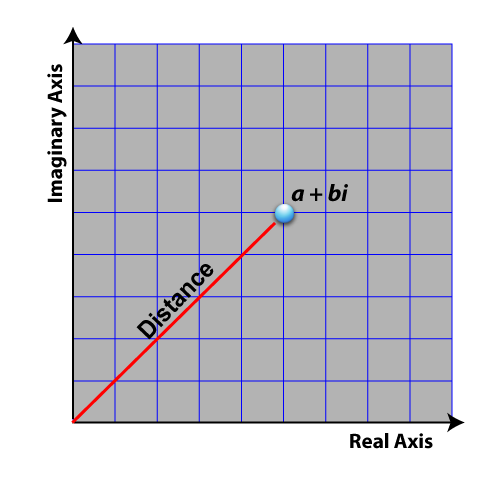This distance is known as the magnitude of the complex number. To learn more about magnitude and comparing complex numbers, click on this link.

 SAT Skill: Working with Imaginary Numbers Example 1 What is the value of this expression if m = 4 and n = 3.Combine the exponents and plug in the values:Recall the repeating pattern starting at an exponent of 1: i, -1, -i, 1. For an exponent of 7, this is the result:Complex Number Operations

In this section we go over the properties of complex numbers, including the following:

• Adding and Subtracting Complex Numbers
• Multiplying and Dividing Complex Numbers
• Closure Property

Adding and subtracting complex numbers involves adding two-dimensional numbers. Remember, a complex number has a real part and an imaginary part. This means that adding or subtracting complex numbers involves real operating on real and imaginary operating on imaginary.To see examples of adding and subtracting complex numbers, click on this link.

Multiplying and Dividing Complex Numbers

When multiplying two complex numbers, use the FOIL method to find the separate products. Then combine real terms and imaginary terms. Note that there will be an i-squared term, that will become a real term.Multiplying a complex number and its conjugate results in a real number, with no imaginary part.Dividing two complex numbers results in another complex number in standard form. However, to get that form, multiply the numerator and denominator by the conjugate of the denominator.To see examples of multiplying and dividing complex numbers, click on this link.

 SAT Skill: Working with Complex Numbers Example 1 What is the sum of these numbers?Combine the real parts and the imaginary parts:Example 2 Subtract these numbers.Combine the real parts and the imaginary parts:Example 3 Combine these numbers.Combine the real parts and the imaginary parts. Note that the i2 term becomes a real number:Complex Roots of Equations

With certain quadratic equations, the solutions to the equations are complex numbers.How do we know when a quadratic equation has complex roots? Calculate the discriminant of the quadratic:If the value of the discriminant is less than zero, then the quadratic will have complex roots.

To see examples of finding the complex roots of quadratic equations, click on this link. This slide show includes a video tutorial and worked-out examples.